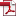• KSII Transactions on Internet and Information Systems
Monthly Online Journal (eISSN: 1976-7277)

# Probabilistic Constrained Approach for Distributed Robust Beamforming Design in Cognitive Two-way Relay Networks

Vol. 12, No. 1, January 30, 201810.3837/tiis.2018.01.002 , Download Paper (Free):#### Abstract

In this paper, we propose the distributed robust beamforming design scheme in cognitive two-way amplify-and-forward (AF) relay networks with imperfect channel state information (CSI). Assuming the CSI errors follow a complex Gaussian distribution, the objective of this paper is to design the robust beamformer which minimizes the total transmit power of the collaborative relays. This design will guarantee the outage probability of signal-to- interference-plus-noise ratio (SINR) beyond a target level at each secondary user (SU), and satisfies the outage probability of interference generated on the primary user (PU) above the predetermined maximum tolerable interference power. Due to the multiple CSI uncertainties in the two-way transmission, the probabilistic constrained optimization problem is intractable and difficult to obtain a closed-form solution. To deal with this, we reformulate the problem to the standard form through a series of matrix transformations. We then accomplish the problem by using the probabilistic approach based on two sorts of Bernstein-type inequalities and the worst-case approach based on S-Procedure. The simulation results indicate that the robust beamforming designs based on the probabilistic method and the worst-case method are both robust to the CSI errors. Meanwhile, the probabilistic method can provide higher feasibility rate and consumes less power.